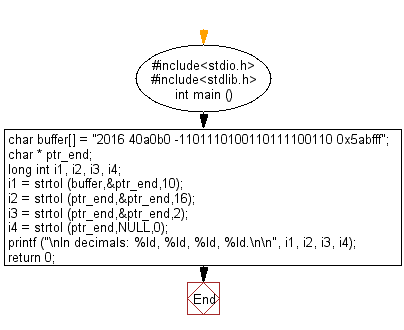﻿ C Program: Convert a string to a long integer - w3resource

# C Exercises: Convert a string to a long integer

## C Variable Type : Exercise-3 with Solution

Write a C program to convert a string to a long integer.

Sample Solution:

C Code:

``````#include<stdio.h>
#include<stdlib.h>

int main ()
{
// Define a character array 'buffer' containing a string of numbers in different bases
char buffer[] = "2016 40a0b0 -1101110100110111100110 0x5abfff";

// Define pointers for strtol function to update
char * ptr_end;

// Define four variables to store converted values
long int i1, i2, i3, i4;

// Convert the first section of 'buffer' to a long integer in base 10
i1 = strtol (buffer, &ptr_end, 10);

// Convert the second section of 'buffer' to a long integer in base 16 (hexadecimal)
i2 = strtol (ptr_end, &ptr_end, 16);

// Convert the third section of 'buffer' to a long integer in base 2 (binary)
i3 = strtol (ptr_end, &ptr_end, 2);

// Convert the fourth section of 'buffer' to a long integer in the appropriate base (auto-detect)
i4 = strtol (ptr_end, NULL, 0);

// Print the converted values in decimal format
printf ("\nIn decimals: %ld, %ld, %ld, %ld.\n\n", i1, i2, i3, i4);

return 0;
}
```
```

Sample Output:

```In decimals: 2016, 4235440, -3624422, 5947391.
```

Flowchart:C Programming Code Editor:

Improve this sample solution and post your code through Disqus

What is the difficulty level of this exercise?

Test your Programming skills with w3resource's quiz.

﻿